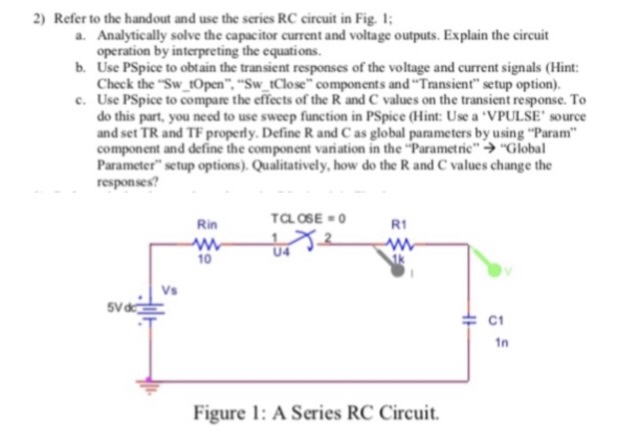# Can you do the PSpice? Thank you 2) Refer to the handout and use the series...

###### Question:

Can you do the PSpice? Thank you2) Refer to the handout and use the series RC circuit in Fig. 1 Analytically solve the capacitor current and voltage outputs.Explain the circuit operation by interpreting the equations Use PSpice to obtain the transient responses of the voltage and current signals (Hint: Check the "Sw tOpen" "Sw tClose" components and Transient" setup option). Use PSpice to compare the effects of the R and C values on the transient response. To do this part, you need to use sweep function in PSpice (Hint: Use a VPULSE' source and set TR and TF properly. Define R and C as global parameters by using "Param" component and define the component variation in the "Parametric" "Global Parameter" setup options).Qualitatively, how do the R and C values change the responses? a. b. e. Rin TCL OSE R1 U4 10 Vs Vd 1n Figure I: A Series RC Circuit.

#### Similar Solved Questions

##### A high voltage power transmission line transmitting 100MW of electrical power is operating at a voltage...
A high voltage power transmission line transmitting 100MW of electrical power is operating at a voltage of 288KV. If the line loses 843KW what is its resistance (give your answer in Ohms)?...
##### QUESTION 10 The population of Kenosha in 2008 was 93,000 people. It has been growing at...
QUESTION 10 The population of Kenosha in 2008 was 93,000 people. It has been growing at a rate of 2% each year. a) Create a mathematical model to represent this situation. b) If the trend continues, how long will it take for the population to reach 150,000?...